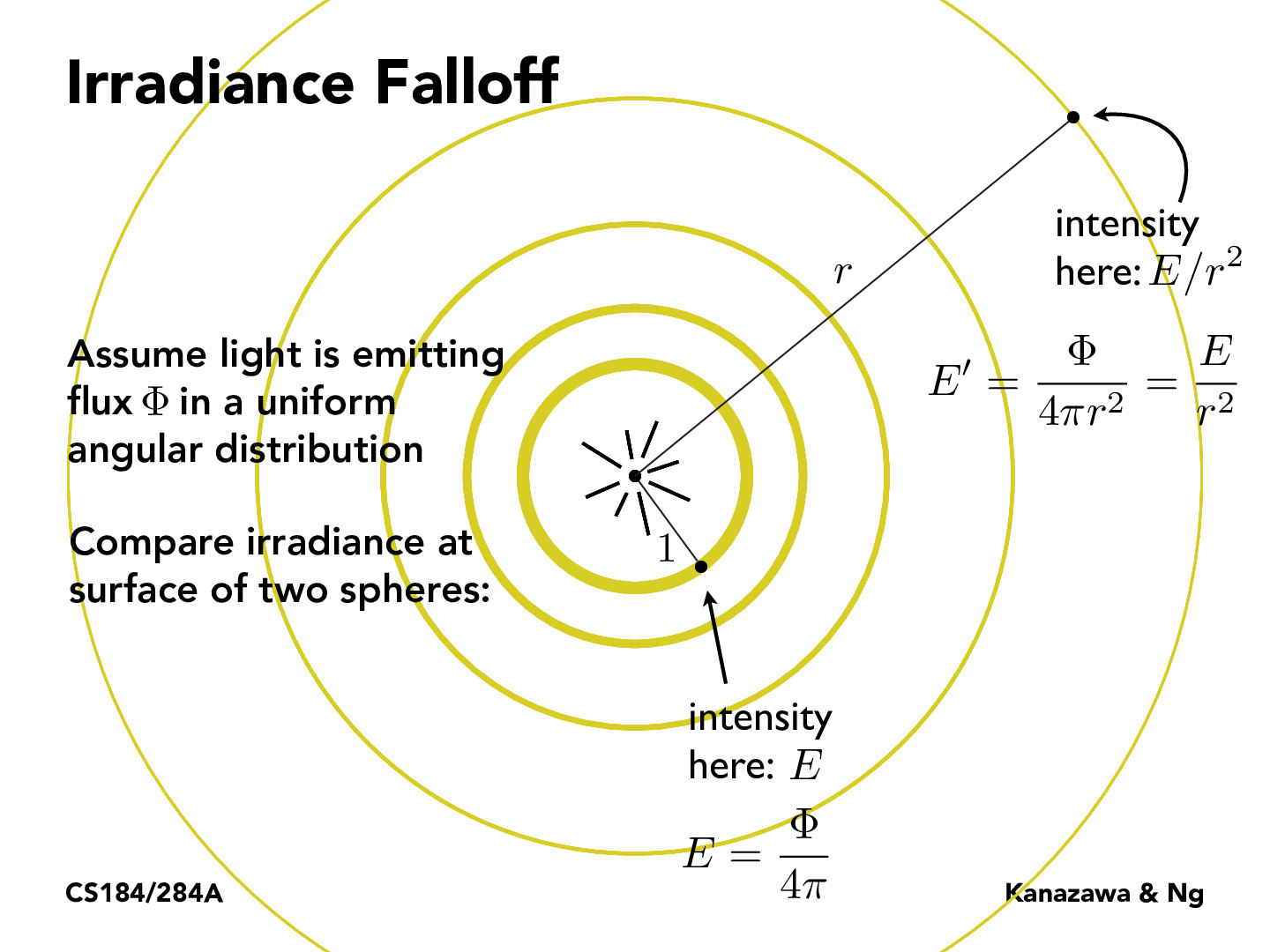Lecture 11: Radiometry & Photometry (29)jerryzhucs21

How would Irradiance calculations change if the flux is not in a uniform angular distribution? It seems that the flux value is a scalar. Is there some way to rewrite these equations in matrix/vector form to reflect non-uniform distribution of light?tancik

@jerryzhucs21 You would use an equation like the one on slide 39. In this case the radiance L_i would be a function dependent on omega, the incoming direction.sethzhao506

This relationship could be referred as an application of inverse-square law, where in a broad way states that a specified physical quantity is inversely proportional to the square of the distance from the source of the physical quantity.nLevin13

@sethzhao506 it seems to be that every physical quantity which travels in a wave through space follows the inverse square law. I wonder if modelling the "intensity" of gravity this way makes sense since it was recently discovered that gravity waves are real.daniswords

I am a bit confused about how to interpret this graphic. What is the 1 meant to represent? Is that some fixed value?

You must be enrolled in the course to comment